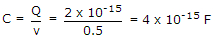# Electronics and Communication Engineering - Digital Electronics

26.

Assertion (A): TTL is a very popular logic in SSI and MSI category.

Reason (R): In Schottky TTL the power dissipation is less than in ordinary TTL.

 A. Both A and R are correct and R is correct explanation of A B. Both A and R are correct but R is not correct explanation of A C. A is true, R is false D. A is false, R is true

Explanation:

In Schottky TTL storage time is reduced.

27.

FF16 when converted to 8421BCD =

 A. 0000 0101 0101 B. 0010 0101 0101 C. 1111 0101 0101 D. 1000 0101 0101

Explanation:

FF in hexadecimal = 15 x 16 + 15 = 255 in decimal = 0010 0101 0101 in BCD.

28.

ECL has high switching speed because the transistors are

 A. switching between cutoff and saturation regions B. switching between cutoff and active regions C. switching between active and saturation regions D. none of the above

Explanation:

High speed is obtained because saturation state is avoided.

29.

Karnaugh map can not be drawn when the number of variables is more than 4

 A. True B. False

Explanation:

K map can be drawn for more than 4 variables.

30.

A dynamic RAM cell which holds 5 V has to be refreshed every 20 ms so that the stored voltage does not fall by more than 0.5 V. If the cell has a constant discharge current of 0.1 pA, the storage capacitance of cell is

 A. 4 x 10-6 F B. 4 x 10-9 F C. 4 x 10-12 F D. 4 x 10-15 F

Q = 20 x 10-3 x 0.1 x 10-12 = 2 x 10-15 C and.